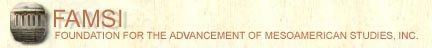John Montgomery
Dictionary of Maya Hieroglyphs

﻿

T

To hear the sounds and learn more, click on the text below the glyph.

Search the dictionary for words in English or Maya.
Please be sure java is enabled on your browser.

A | B' | CH | CH' | E | H | I | J | K | K' | L | M | N | O | P | S | T | T' | TZ | TZ' | U | W | X | Y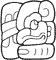TA OCH-le-{l} (ta ochlel)
(T102.765:188)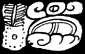TA yu-ta-{l} (ta yutal)
(T51.61:565)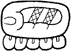ta-ji (taj)
(T565v:136)ta-ki (-tak)
(T565v.102)ta-ki-ja (takaj)
(T565:102.181)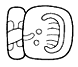ta-li (tal)
(T113.82)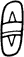ta/TA (ta)
(T103)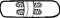ta/TA (ta)
(T113)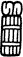ta/TA (ta)
(T122:150)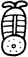ta/TA (ta)
(T51 and T53)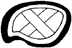ta/TA (ta)
(T552v)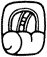ta/TA (ta)
(T565)ta/TA (ta)
(T565)ta/TA (ta)
(T565)ta/TA (ta)
(T645)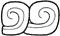ta/TA (ta)
(T776.776)TAJ (taj)
(T122:150)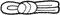TAJ (taj)
(T60)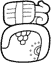TAJ TUN (taj tun)
(T122.150:528)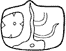TAK (-tak)
(T559)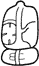TAK-ki (-tak)
(T559:102)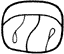TAL (tal)
(T676)TAN (tan)
(T606v)TAN (tan)
(T606)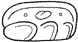TAN (tan)
(T606)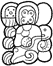TAN CH'EN OX WITZ-aj (tan ch'en ox witzaj)
(T606.571v:III.528hv.228)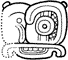TAN CH'EN-na (tan ch'en)
(T606.598v:23)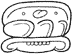TAN-na (tan)
(T606:23)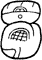te'-k'a (tek')
(T580:669)te'-k'a-ja (te' k'aj)
(T580:669b.181)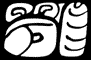TE'-le (te'el)
(T87:513.188)te/TE'/CHE' (te/te'/che')
(T350)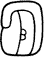te/TE'/CHE' (te/te'/che')
(T513)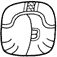te/TE'/CHE' (te/te'/che')
(T514)te/TE'/CHE' (te/te'/che')
(T514v)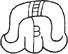te/TE'/CHE' (te/te'/che')
(T514v)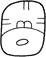te/TE'/CHE' (te/te'/che')
(T580)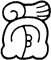te/TE'/CHE' (te/te'/che')
(T78:514v)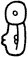te/TE'/CHE' (te/te'/che')
(T87)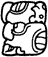te/TE'/CHE' (te/te'/che')
(T87hv)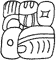TI AJAW le-{l} (ti ajawlel)
(T59.168:188)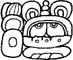TI ya-AK'-il (ti yakil?)
(T59.126:504v:24)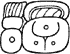ti-ka-la (tikal)
(T59.25:534)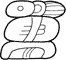ti-ki-li (tikil)
(T59:102:24)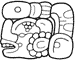ti-xu-[ku]-pi (ti xukpi)
(T59:756.177)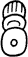ti/TI (ti)
(T59)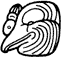ti/TI (ti)
(T747)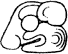TIL (til)
(T758v)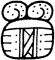to (to)
(T44:563b)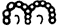to (to)
(T44)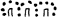to (to)
(T44)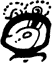to-jo-li (tojol)
(T44:607:24v)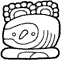to-jo-li (tojol)
(T44:695:24)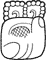to-k'a (tok')
(T44:669:314)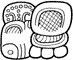to-k'a pa-ka-la (tok' pakal)
(T44:669.586b:25:178)to-ko (tok)
(T44:110)to-ko TAN AJAW (Tok Tan Ajaw)
(T110.168:44:606)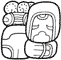to-ko TAN WINIK-ki (Tok Tan Winik)
(T44:110:606.521:102)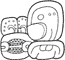to-ko-TAN-na (tok tan/Tok Tan)
(T44:110.606:23)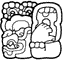to-XAT (toxat)
(T1030j)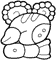to-[xa]-AT-ti (toxat)
(T44:691v:59)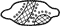TOK' (tok')
(T257)TOK' (tok')
(T257)TOK' (tok')
(T257)TOK' PAKAL (tok' pakal)
(T257:624)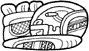TU K'U-li (tuk'ul)
(T89.1016:24)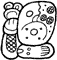TU WAY-[b'i]-li (tu wayb'il)
(T89.539:82)tu-ku-nu (tukun)
(T89.528:592v)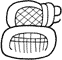tu-pa (tup)
(T89:586)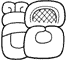tu-pa-ja (tupaj)
(T89.586:683)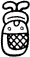tu/TU (tu)
(T89, T90, and T92)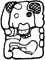TUN (tun)
(T1034)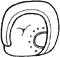TUN (tun)
(T528)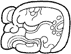TUN (tun)
(T528hv)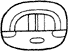TUN (tun)
(T548)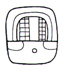TUN (tun)
(T548)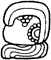TUN-ni (tun)
(T528:116)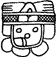[TAN] LAM (tan lam)
(T699v)[TAN] LAM-ja (tan lamaj)
(T699v.181)
Index | Next Page
Return to top of page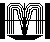Order John Montgomery’s Book